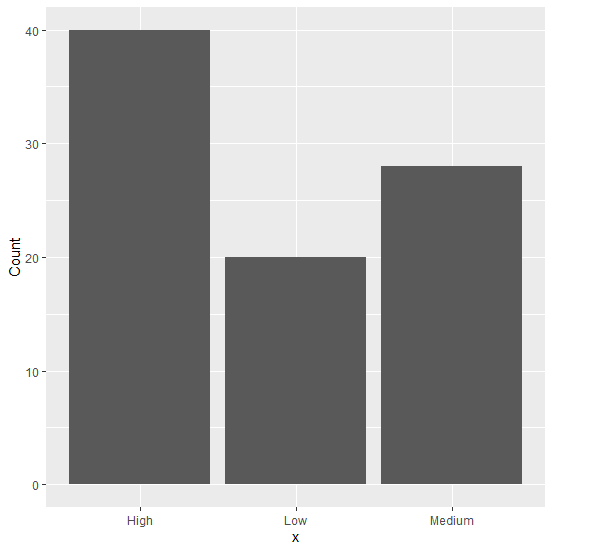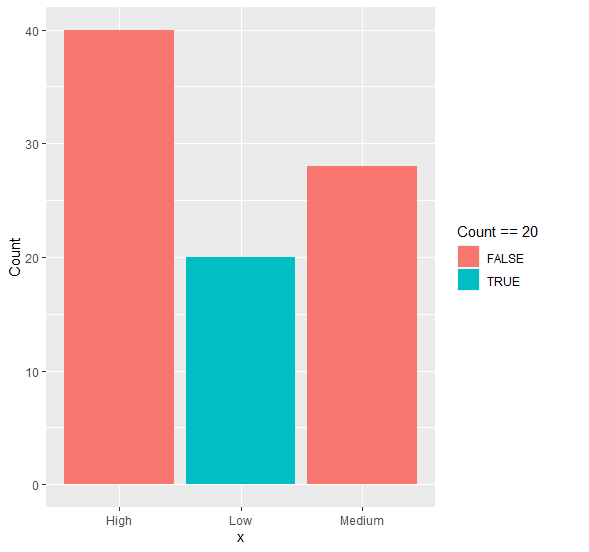# How to change the color of a particular bar using geom_bar in R?

To change the color of a particular bar using geom_bar in R, we can provide the count corresponding to the value for which we want to change the color inside aes function.

For Example, if we have a data frame called df that contains two columns say V and F where V is categorical and F is for frequency and we want to change the color of frequency 10 in bar plot then we can use the below mentioned command −

ggplot(df,aes(V,F))+geom_bar(aes(fill=..F..==10),stat="identity")

## Example

Following snippet creates a sample data frame −

x<-c("Low","Medium","High")
Count<-c(20,28,40)
df<-data.frame(x,Count)
df

The following dataframe is created

    x  Count
1 Low    20
2 Medium 28
3 High   40

To load ggplot2 package and create bar plot for data in df on the above created data frame, add the following code to the above snippet −

x<-c("Low","Medium","High")
Count<-c(20,28,40)
df<-data.frame(x,Count)
library(ggplot2)
ggplot(df,aes(x,Count))+geom_bar(stat="identity")

## Output

If you execute all the above given snippets as a single program, it generates the following Output −To create bar plot for data in df with bar in different color having Count = 20 on the above created data frame, add the following code to the above snippet −

x<-c("Low","Medium","High")
Count<-c(20,28,40)
df<-data.frame(x,Count)
library(ggplot2)
ggplot(df,aes(x,Count))+geom_bar(aes(fill=..Count..==20),stat="identity")

## Output

If you execute all the above given snippets as a single program, it generates the following Output −